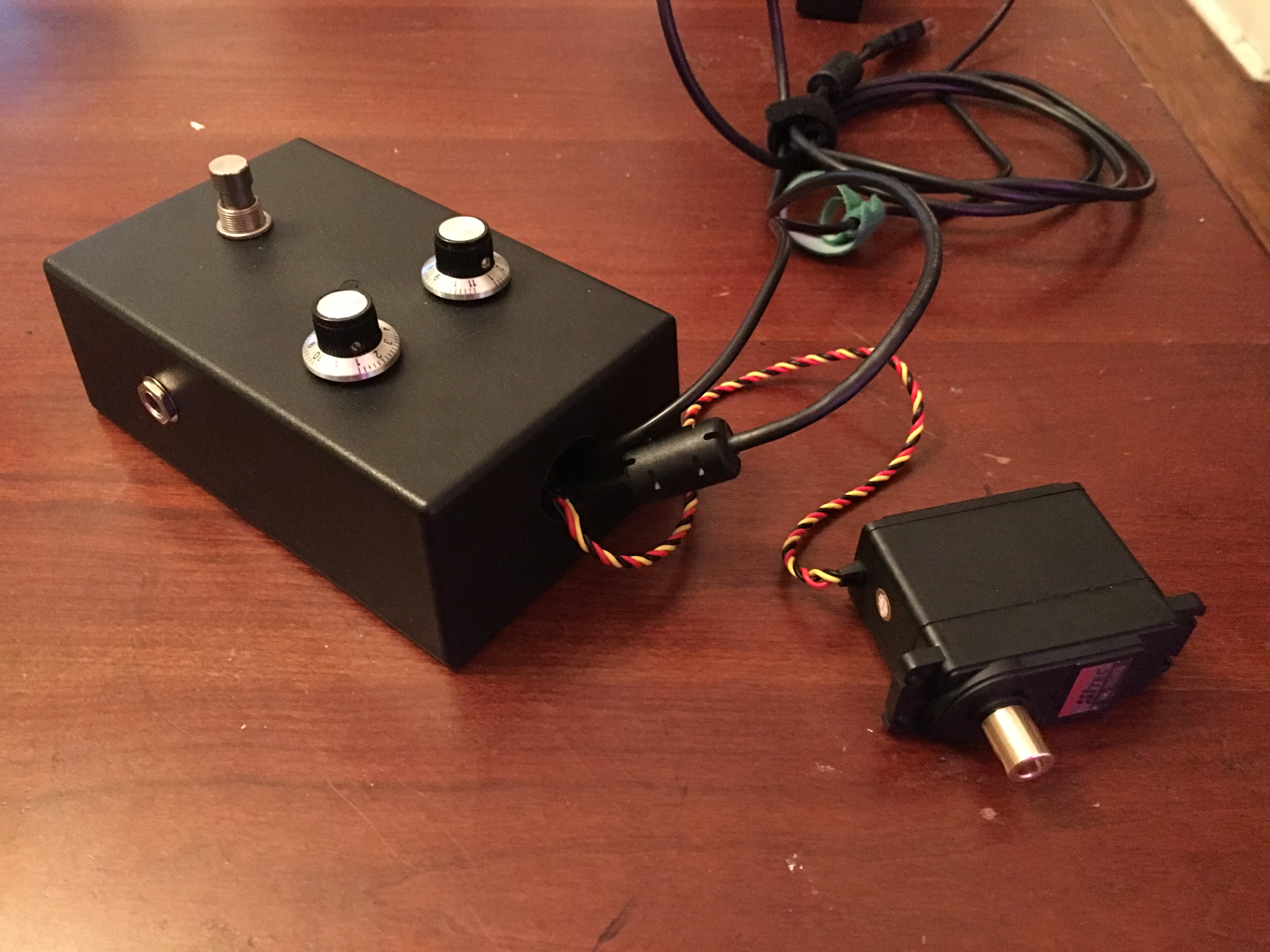# The Expressifier - Wired Version

Over the course of this build, I kept running into issues with my wireless units. So in order to get a working prototype done, I scratched the wireless component of this project for the meantime and focused on making a single module. I used a pre-made plastic enclosure and added the components and then made a simple servo mount out of wood.The Arduino code is posted below:

``````#include <Servo.h>

Servo myservo;
const int numReadings = 10;

int pos;
int low = 0;
int high = 22;
int readings[numReadings];      // the readings from the analog input
int readIndex = 0;              // the index of the current reading
int total = 0;                  // the running total
int average = 0;                // the average

int inputPin = A0;

void setup() {
pinMode(2, INPUT);
myservo.attach(9);
// initialize serial communication with computer:
Serial.begin(9600);
// initialize all the readings to 0:
for (int thisReading = 0; thisReading < numReadings; thisReading++) {
readings[thisReading] = 0;
}
}

void loop() {
if (digitalRead(2) == HIGH){
// subtract the last reading:
total = total - readings[readIndex];
// read from the sensor:
readings[readIndex] = analogRead(inputPin);
// add the reading to the total:
total = total + readings[readIndex];
// advance to the next position in the array:
readIndex = readIndex + 1;

// if we're at the end of the array...
if (readIndex >= numReadings) {
// ...wrap around to the beginning:
readIndex = 0;
}

// calculate the average:
average = total / numReadings;
// send it to the computer as ASCII digits
low = map (analogRead(A1), 0, 1024, 180, 0);
high = map (analogRead(A2), 0, 1024, 180, 0 );
Serial.println(high);
pos = map (average, 0, 1023, low, high);
//Send the message:
myservo.write(pos);
delay(1);        // delay in between reads for stability
}
}
``````
Written on December 5, 2017